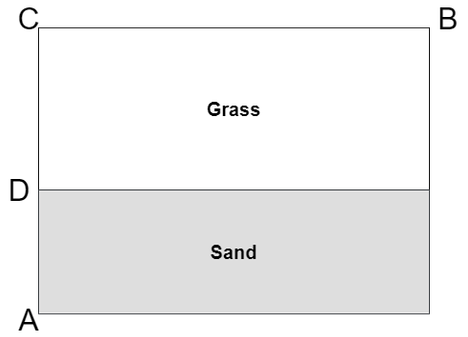# Practice on Toph

Participate in exhilarating programming contests, solve unique algorithm and data structure challenges and be a part of an awesome community.

# Why the Long Face?

By ishtupeed · Limits 1s, 512 MB

Consider the following figure:Ciri and her horse Kelpie are standing at point $A$. She needs to go to a coffee bar located at point $B$. In front of them are the sand region (the shaded region) with width $AD$ and the grass region with width $CD$. Assume that both the grass region and the sand region extend towards infinity with the same respective width in both directions. Kelpie can go twice as fast over the grass than the sand. Ciri wants to ride her horse to reach $B$ as fast as possible.

Given the length of $BC$, $CD$, and $AD$, find out the length of the path that Ciri and Kelpie will take.

## Input

The first line of the input will contain an integer $T (1 \leq T \leq 100)$, denoting the number of test cases.

For each test case, there will be three integers, $BC$, $CD$, and $AD (0 < BC, CD, AD \leq 100)$ - as described in the problem statement.

## Output

For each test case, print a single value denoting the length of the path followed by a newline. Absolute errors less than $10^{-4}$ will be ignored.

## Sample

InputOutput
1
40 31 21

67.0000


### Statistics

82% Solution Ratio

BrehamPieEarliest, 1M ago

NJRafiFastest, 0.0s

BrehamPieLightest, 131 kB

SyedshakilmahmShortest, 526B

### Submit